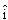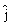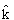# Vectors Multiple Choice Questions### Vector quiz Physics:

Ques. If more than three forces are acting on the massive point object, then the object will be in equilibrium if and only if all the forces are
(a) collinear
(b) coplanar
(c) acting in random directions
(d) represented by the sides of a closed polygon

Ans. (d)

Ques. A man crosses a 320 m wide river perpendicular to the current in 4 minutes. If in still water he can swim with a speed 5/3 times that of the current, then the speed of the current, in m/min is
(a) 30
(b) 40
(c) 50
(d) 60

Ans. (d)

Ques. Two forces of 200 N and 120 N are inclined at an angle of 60o. The resultant force will be
(a) 40 N
(b) 180 N
(c) 280 N
(d) 340 N

Ans. (c)

Ques. The sum of magnitudes of two forces acting at a point is 16 and magnitude of their resultant is 8√3. If the resultant is at 90o with the force of smaller magnitude, then their magnitude are :
(a) 3, 13
(b) 2, 14
(c) 5, 11
(d) 4, 12

Ans. (b)

Ques. Can the resultant of 2 vectors be zero?
(a) Yes, when the 2 vectors are same in magnitude and direction
(b) No
(c) Yes, when the 2 vectors are same in magnitude but opposite in sense
(d) Yes, when the 2 vectors are same in magnitude making an angle of 2/3 with each other

Ans. (c)

Ques. A boat crosses a river with a velocity of 8 km/h. If the resulting velocity of boat is 10 km/h then the velocity of river water is
(a) 4 km/h
(b) 6 km/h
(c) 8 km/h
(d) 10 km/h

Ans. (b)

Ques. A particle has two equal accelerations in two given directions. If one of the accelerations is halved, then the angle which the resultant makes with the other is also halved. The angle between the accelerations is
(a) 120o
(b) 90o
(c) 60o
(d) 45o

Ans. (a)

Ques. A river is flowing from east to west at a speed of 5 m/min. A man on south bank of river, capable of swimming 10m/min in still water, wants to swim across the river in shortest time. He should swim
(a) Due north
(b) Due north-east
(c) Due north-east with double the speed of river
(d) None of these

Ans. (a)

Ques. A man sitting in a bus travelling in a direction from west to east with a speed of 40 km/h observes that the rain-drops are falling vertically down. To the another man standing on ground the rain will appear
(a) To fall vertically down
(b) To fall at an angle going from west to east
(c) To fall at an angle going from east to west
(d) The information given is insufficient to decide the direction of rain.

Ans. (b)

Ques. In the above problem, the speed of raindrops w.r.t. the moving man, will be
(a) 10√2 km/h
(b) 5 km/h
(c) 10√3 km/h
(d) 5/√3 km/h

Ans. (c)

Ques. The vectors 5i + 8j and 2i + 7j are added. The magnitude of the sum of these vector is
(a) √274
(b) 38
(c) 238
(d) 560

Ans. (a)

Ques. Two equal vectors have their resultant equal to three fourth of either of them. The angle between them is
(a) 44o
(b) 51.3o
(c) 136o
(d) 128.7o

Ans. (c)

Ques. A thief is running away on a straight road on a jeep moving with a speed of 9 m/s. A police man chases him on a motor cycle moving at a speed of 10 m/s. If the instantaneous separation of jeep from the motor cycle is 100 m, how long will it take for the policemen to catch the thief
(a) 1 second
(b) 19 second
(c) 90 second
(d) 100 second

Ans. (d)

Ques. y component of velocity is 20 and x component of velocity is 10. The direction of motion of the body with the horizontal at this instant is
(a) tan–1 (2)
(b) tan–1 (1/2)
(c) 45°
(d) 0°

Ans. (a)

Related: Solid state chemistry questions

Ques. A body, acted upon by a force of 50 N is displaced through a distance 10 meter in a direction making an angle of 60° with the force. The work done by the force be
(a) 200 J
(b) 100 J
(c) 300 J
(d) 250 J

Ans. (d)

Ques. Two forces 3N and 2 N are at an angle θ such that the resultant is R. The first force is now increased to 6N and the resultant become 2R. The value of θ is
(a) 30°
(b) 60°
(c) 90°
(d) 120°

Ans. (d)

Ques. Two vectors have their resultant equal to either of them. The angle between them is
(a) 45o
(b) 75o
(c) 90o
(d) 120o

Ans. (d)

Ques. A scooter going due east at 10 ms–1 turns right through an angle of 90°. If the speed of the scooter remains unchanged in taking turn, the change is the velocity of the scooter is
(a) 20.0 ms–1 south eastern direction
(b) Zero
(c) 10.0 ms–1 in southern direction
(d) 14.14 ms–1 in south-west direction

Ans. (d)

Ques. Two forces, F1 and F2 are acting on a body. One force is double that of the other force and the resultant is equal to the greater force. Then the angle between the two forces is
(a) cos–1 (1/2)
(b) cos–1 (–1/2)
(c) cos–1 (–1/4)
(d) cos–1 (1/4)

Ans. (c)

Ques. A plane is revolving around the earth with a speed of 100 km/hr at a constant height from the surface of earth. The change in the velocity as it travels half circle is
(a) 200 km/hr
(b) 150 km/hr
(c) 100√2 km/hr
(d) 0

Ans. (a)

Ques. For the resultant of the two vectors to be maximum, what must be the angle between them
(a) 0°
(b) 60°
(c) 90°
(d) 180°

Ans. (a)

Ques. If the sum of two unit vectors is a unit vector, then magnitude of difference is
(a) √2
(b) √3
(c) 1/√2
(d) √5

Ans. (b)

Ques. A truck travelling due north at 20 m/s turns west and travels at the same speed. The change in its velocity be
(a) 40 m/s N–W
(b) 20√2 m/s N–W
(c) 40 m/s SW
(d) 20√2 m/s SW

Ans. (d)

Ques. Five equal forces of 10 N each are applied at one point and all are lying in one plane. If the angles between them are equal, the resultant force will be
(a) Zero
(b) 10 N
(c) 20 N
(d) 10√2N

Ans. (a)

Ques. The magnitude of a given vector with end points (4, – 4, 0) and (– 2, – 2, 0) must be
(a) 6
(b) 5√2
(c) 4
(d) 2√10

Ans. (d)

Ques. The sum of the magnitude of two forces acting at a point is 18 and the magnitude of their resultant is 12. If the resultant is at 90o with the force of the smaller magnitude, then their magnitudes are
(a) 3, 15
(b) 4, 14
(c) 5, 13
(d) 6, 12

Ans. (c)

Ques. Let u = i + j, v = i – j and w = i + 2j + 3k. If n is a unit vector such that u.n = 0 and v.n = 0 then | w . n | is equal to
(a) 0
(b) 1
(c) 2
(d) 3

Ans. (d)

Ques. A force of 5 N acts on a particle along a direction making an angle of 60° with vertical. Its vertical component be
(a) 10 N
(b) 3 N
(c) 4 N
(d) 2.5 N

Ans. (d)

Related: Coordinate geometry online test

Ques. 100 coplanar forces each equal to 10 N act on a body. Each force makes angle π/50 with the preceding force. What is the resultant of the forces
(a) 1000 N
(b) 500 N
(c) 250 N
(d) Zero

Ans. (d)

Ques. If the difference of two unit vectors is again a unit vector, then the angle between them is
(a) 30º
(b) 40º
(c) 60º
(d) 90º

Ans. (c)

Ques. A force of 10 N acts on a particle along a direction making an angle of 60o with vertical. What is the component of the force in the vertical direction ?
(a) 10 N
(b) 5 N
(c) 2 N
(d) 1 N

Ans. (b)

Ques. The divergence of the vector field 3xz+ 2xy– yz2at a point (1,1,1 ) is equal to
(a) 7
(b) 4
(c) 3
(d) 0

Ans. (c)